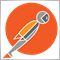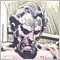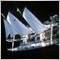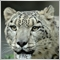# Trying to "Normalize Double" but it returns the same precision as the original value2124

I'm getting an RSI value of 64.569404663319915

So I tried NormalizeDouble(rsiValue, 1) but that didn't work

So then I made my own function:

```double roundNearest(double value, int digits) {
double base10 = MathPow(10,digits);

value *= base10;
value = MathRound(value);
value /= base10;

return value;
} ```

It works up until I have to divide by base10, but then it still gives me the same precision as the original RSI value.

How can I go about getting the precision I want?

Thanks.2392

You have to use DoubleToString when printing to log.2124

nicholi shen:
You have to use DoubleToString when printing to log.
Thanks, although I'm interested in knowing how to normalize a double outside of printing to the log or converting to string.3251

Alexander Martinez:

It works up until I have to divide by base10, but then it still gives me the same precision as the original RSI value.

Because you aren't doing anything to truncate the value once you've rounded it.

This may help: https://www.mql5.com/en/forum/268708#comment_8204877

Note how I use the (long) typecast to chop off the decimal side of the double.2124

Anthony Garot:

Because you aren't doing anything to truncate the value once you've rounded it.

This may help: https://www.mql5.com/en/forum/268708#comment_8204877

Note how I use the (long) typecast to chop off the decimal side of the double.

Thanks for the response.

I tried your code out, but I see that you're rounding to the nearest whole number.

I'm trying to round to the specified precision. For example, in the code I posted in my OP, 64.569404663319915 should round to 64.6.3251

Alexander Martinez:

Thanks for the response.

I tried your code out, but I see that you're rounding to the nearest whole number.

I'm trying to round to the specified precision. For example, in the code I posted in my OP, 64.569404663319915 should round to 64.6.

My code doesn't round at all. That wasn't it's intention.

As I said:

"Note how I use the (long) typecast to chop off the decimal side of the double."

That's the piece of the puzzle you need to correct your code.533

Hi Alexander...

Don't try to round it.... just create 2 variables... 1 - store the value and then the 2nd - to Normalize... something like this:

```double RSI_Value,
RSI_Normalized;
RSI_Value = (iCustom(Symbol(),0,"Rhythm_RSI_Indi",RSIPeriod,0,i));
RSI_Normalized = NormalizeDouble(RSI_Value,1);  // or 2 or whatever decimal you want```

...or you can just go straight:

`RSI_Value = NormalizeDouble((iCustom(Symbol(),0,"Rhythm_RSI_Indi",RSIPeriod,0,i)),1);`2124

Thanks for the help guys, but I'm afraid it's not working.

Mike, I tried your solution, but it doesn't set the precision to digits in NormalizeDouble.

Anthony, apologies, I was a bit burnt out yesterday. Anyway, I tried again today and, unless I'm not understanding you, it's just not working.

Here's what I think Anthony's proposed solution should look like on my end, with all the steps outlined, and also for your convenience if you want to copy + paste it into your editor and debug:

```double roundNearest(double value, int digits) {
double base10  =  MathPow(10,digits);
double a       =  value * base10;
double b       =  MathRound(value * base10);
long c         =  (long) b;
double d       =  c / base10;
return d;
}```

the input parameters are:

`roundNearest(64.569404663319915,1);`

Expected result is:

`64.6`

Actual result is:

`64.5999999999999943`

To be clear, the function should round to the nearest decimal as specified in digits and set the double precision  to what is specified in digits.

Thanks.Moderator
20312

Please refer to the documentation for NormalizeDouble().

"Please note that when output to Journal using the Print() function, a normalized number may contain a greater number of decimal places than you expect."

The number 64.6 will not be stored in your computer as the precise number, it will always be stored as something like 64.59??????????

Normalizing the number will not change the way it is output in the Print() function. You will need to use DoubleToString().

Even if you don't understand why it is stored this way, it is important that you accept it.2124

Keith Watford:

Please refer to the documentation for NormalizeDouble().

"Please note that when output to Journal using the Print() function, a normalized number may contain a greater number of decimal places than you expect."

The number 64.6 will not be stored in your computer as the precise number, it will always be stored as something like 64.59??????????

Normalizing the number will not change the way it is output in the Print() function. You will need to use DoubleToString().

Even if you don't understand why it is stored this way, it is important that you accept it.

Like I mentioned earlier, I'm not outputting to to Print(), unless the Debugger window is the same as Print()? Also, if I compare 64.6 to 64.5999999999999943, will they be the same?

Edit: just tried it out, they are equal.882

Hello friend,

I actually prefer my programs to read data "as is"

After data is received, I make a decision to modify its actual value. I normally don't change it before its been read.